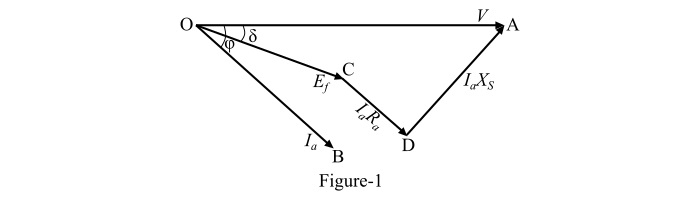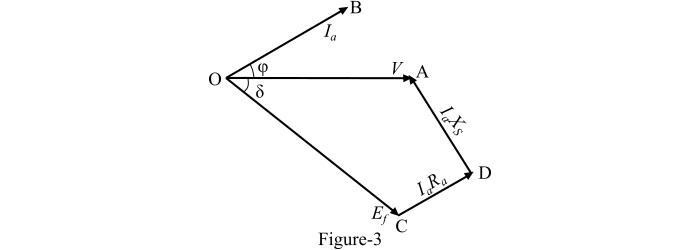# Phasor Diagrams of a Cylindrical Rotor Synchronous Motor

Let,

• Ef=Excitation voltage

• V= Terminal voltage per phase applied to the armature

• Ia=Armature current per phase drawn by the motor from the supply

• Ra= Effective armature resistance per phase

• XS=Synchronous reactance per phase of armature winding

• Cosφ= Power factor

• δ=Torque angle

The voltage equation of a cylindrical rotor synchronous motor is,

$$\mathrm{V=E_{f}+I_{a}(R_{a}+jX_{S})\:\:\:\:\:\:...(1)}$$

$$\mathrm{\Longrightarrow\:V=E_{f}+I_{a}R_{a}+jI_{a}X_{S}\:\:\:\:\:\:...(2)}$$

The phasor diagrams of a 3-phase cylindrical rotor synchronous motor operating at different power factors can be drawn with the help of eqn.(2).

## Phasor Diagram at Lagging Power Factor

The phasor diagram of the synchronous motor operating at a lagging power factor Cosφ is shown in Figure-1.Consider the synchronous motor is taking a lagging current from the supply. Here, the supply voltage (V) is taken as the reference phasor along OA such that OA = V. For lagging power factor cosφ, the armature current (Ia) lags behind the supply voltage (V) by an angle φ along OB where OB = Ia.

The voltage drop in the armature resistance is IaRa which is in phase with the armature current. The phasor IaRa is represented by CD.

The voltage drop per phase in the synchronous reactance is IaXS .The phasor is in a direction perpendicular to the phasor IaRa and is represented by DA.

Therefore, the phasor V is equal to the phasor sum of Ef, and IaRa. The angle δ between V and Ef is called the torque angle. It plays an important role in the power transfer and the stability of the synchronous motor operation.

## Phasor Diagram at Unity Power Factor

The phasor diagram of the synchronous motor operating at unity power factor is shown in Figure-2.Suppose that the synchronous motor is drawing the current (Ia) from the supply at unity power factor. Here, the supply voltage (V) is taken as the reference phasor along OA such that OA = V.

For unity power factor, the armature current (Ia) drawn by the motor is in phase with the supply voltage (V) and is represented by OB where OB = Ia.

The voltage drop in the armature resistance is IaRa which is in phase with the armature current. The phasor IaRa is represented by CD.

The voltage drop per phase in the synchronous reactance is IaXS. The phasor is IaXS in a direction perpendicular to the phasor IaRa and is represented by DA.

Therefore, the phasor V is equal to the phasor sum of Ef,IaRa and jIaXS.The angle δ between V and Ef is called the torque angle. It plays an important role in the power transfer and the stability of the synchronous motor operation

## Phasor Diagram at Leading Power Factor

The phasor diagram of the synchronous motor operating at leading power factor cosφ is shown in Figure-3.Suppose that the synchronous motor is drawing the current (Ia) from the supply at leading power factor cosφ. Here, the supply voltage (V) is taken as the reference phasor along OA such that OA = V.

For leading power factor, the armature current(Ia) drawn by the motor leads the supply voltage (V) by the phase angle φ and is represented by OB where OB = Ia.

The voltage drop in the armature resistance is IaRa which is in phase with the armature current. The phasor IaRa is represented by CD.

The voltage drop per phase in the synchronous reactance isIaXS. The phasor is IaXS in a direction perpendicular to the phasor IaRa and is represented by DA.

Therefore, the phasor V is equal to the phasor sum of Ef,IaRa and jIaXS.